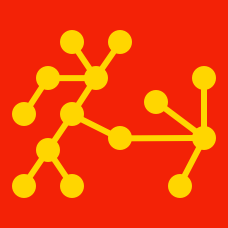Probability

# Graph Theory: Level 1 Challenges

Cosmic liaisons are established among the nine planets of the solar system. Rockets can only travel along the following routes: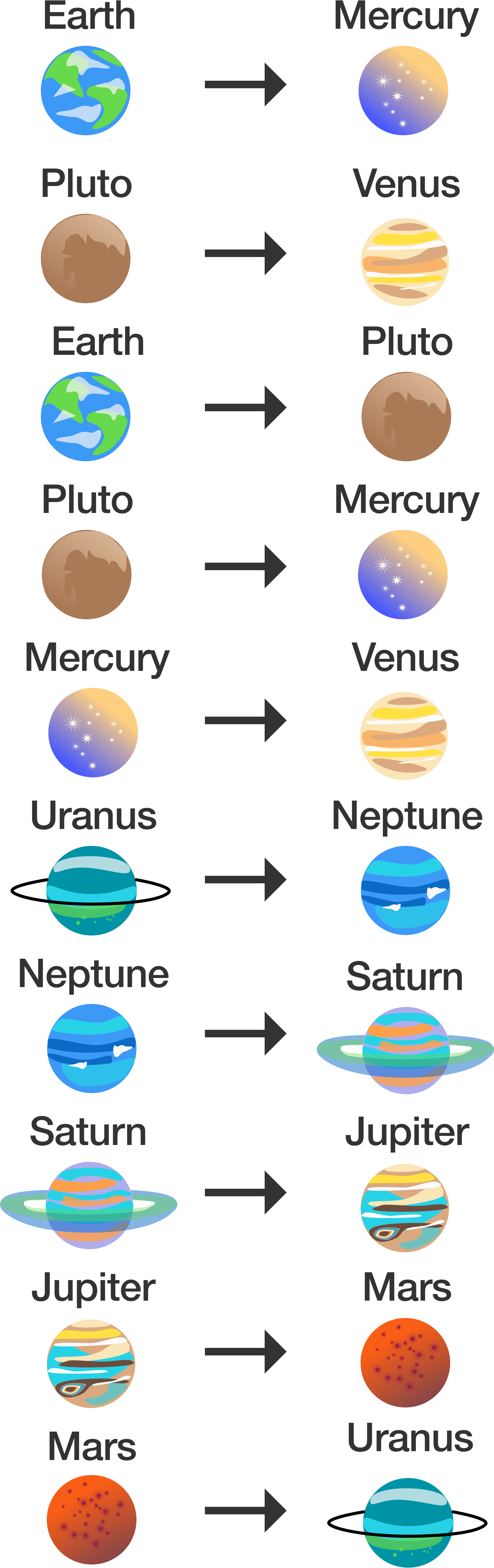Can a traveler get from Earth to Mars?

Five cities $P,Q,R,S,T$ are connected by different modes of transport as follows

• $P$ and $Q$ connected by boat as well as rail.
• $S$ and $R$ connected by bus and boat.
• $Q$ and $T$ connected by air only.
• $P$ and $R$ connected by boat only.
• $T$ and $R$ connected by rail and bus.

If a person visits each of the places starting from $P$ and gets back to $P,$ which of the following places must he visit twice?

What is the least number of colors needed to color each vertex of the graph below such that no two adjacent vertices have the same color?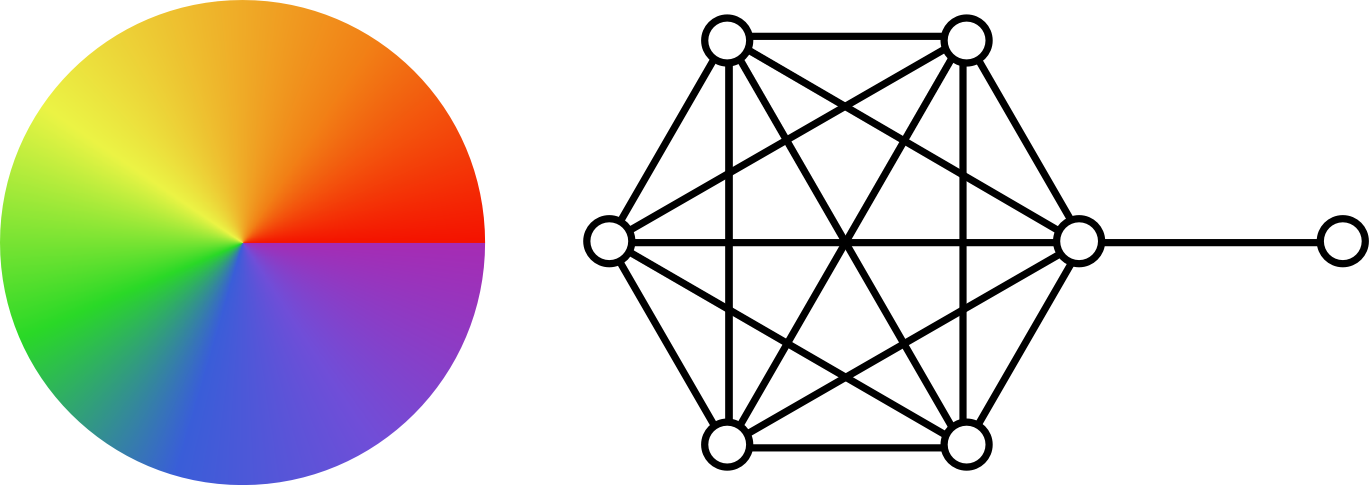Note: In graph theory, two vertices are adjacent if they are connected by an edge.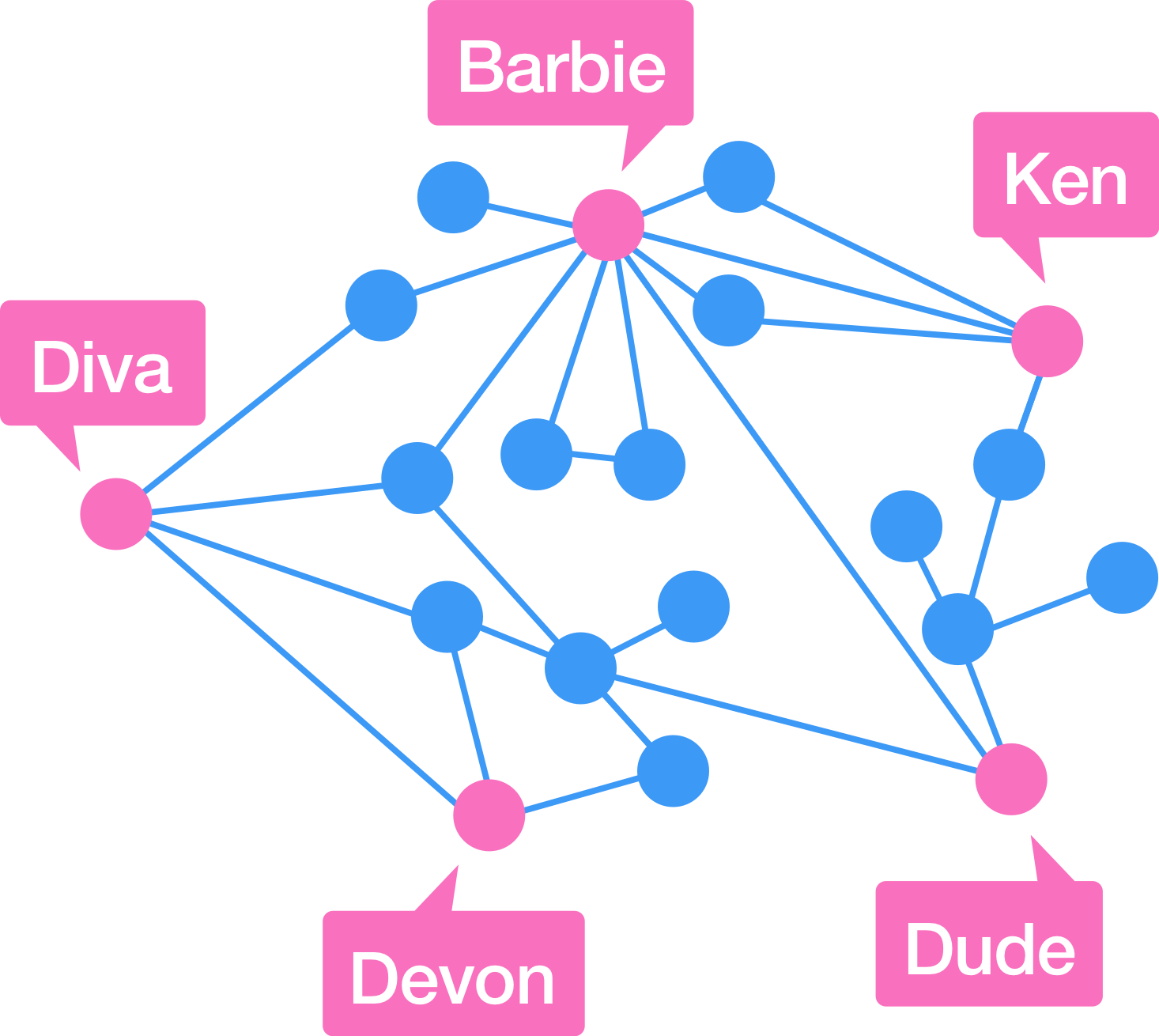Here is a network graph constructed with data from Facebook of 20 people and all of the mutual friendship connections among them. Clearly Barbie has the most friends to invite to her parties (9), but if invitations go out not only to friends but also to all friends of friends, then whose party will have the most invites?

Notes and assumptions

• Each dot represents a person.
• Each line represents mutual friendship between the people on either end .

Which of the shapes above cannot be traced without lifting up your pencil and without tracing over the same edge twice?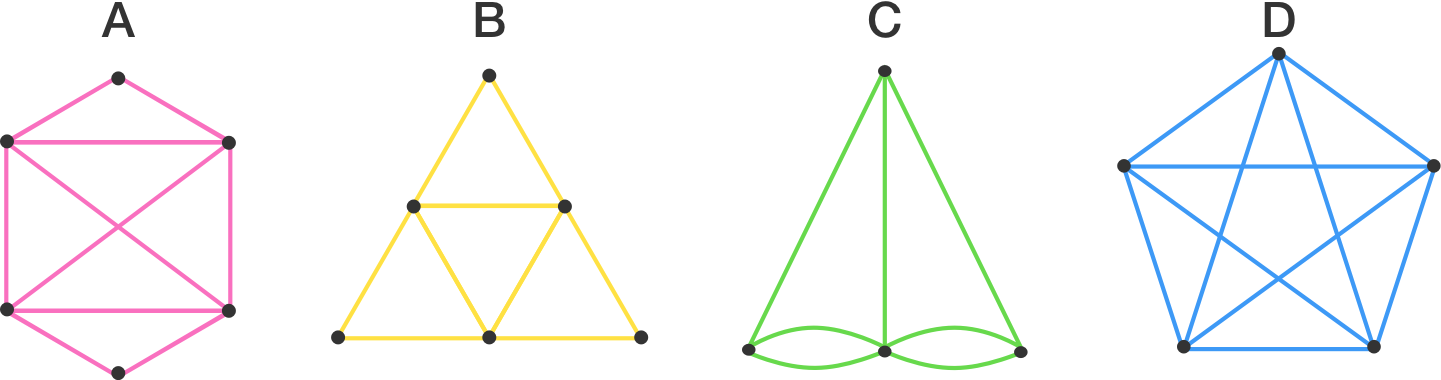×